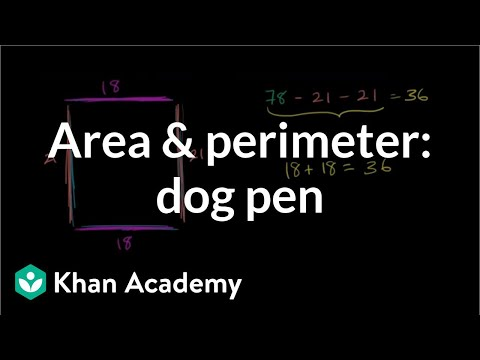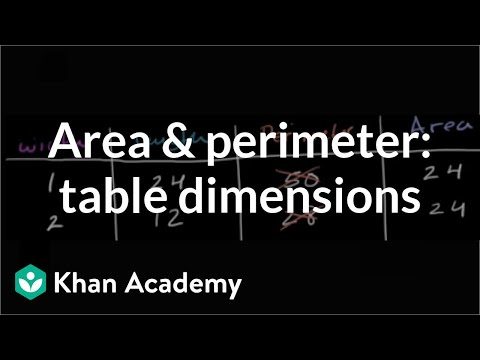Video

# Transitioning from unit squares to area formula (Full video)

Description: Lindsay finds the area of a rectangle both by counting unit squares and multiplying side lengths. This square is 1 square unit, so what is the area of Rectangle A? The first thing we're told is that each of these little squares equal 1 square unit, and then, we're asked to find the area of Rectangle A. Here's Rectangle A, and area is the space that it covers.

### Other videos you might be interested in### Area & perimeter word problem: dog pen (Full video)